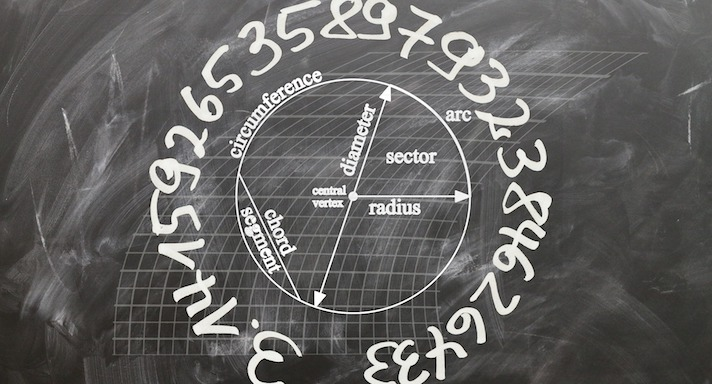# Trigonometry### Course Overview

In Acellus Trigonometry, students learn about the relationships between the sides and the angles of triangles and how to make calculations based on them using trigonometric functions.  Acellus Trigonometry is taught by award-winning Acellus Master Teacher, Patrick Mara. Acellus Trigonometry is A-G Approved through the University of California.

### Course Objectives & Student Learning Outcomes

Upon successful completion of Acellus Trigonometry, students will have attained a mastery of the trig foundational skills necessary for success in higher mathematics. Students will have mastered the unit circle, memorizing the coordinates of various key angles to quickly determine the lengths of the sides of common right triangles. Students will know how to use the sine, cosine, tangent and their reciprocal and inverse functions to determine unknown sides and angles of right triangles. They know what the graphs of these functions look like and how to translate them. They will know how to calculate arc length and sector area. Students will be confident using various trig formulas, such as the Law of Sines and the Law of Cosines, as well as the area formula for triangles. They also are familiar with and know how to use the trig identities. Students are familiar working with vectors and know how to calculate magnitude and direction from horizontal and vertical components and vice versa. They also know how to add vectors both geometrically and algebraically. Students know how to solve trig equations. Throughout this course, students gain experience using trigonometry to solve problems based on real-world situations.This course was developed by the International Academy of Science. Learn More

### Scope and Sequence

Unit 1 – Preliminaries This unit covers similarity and proportion, 30-60-90 and 45-45-90 right triangles, rationalizing the denominator, degrees, minutes, and seconds. Unit 2 – Right Triangle Trigonometry This unit discusses sine, cosine and tangent, word problems for each, as well as pythagorean and tangent identities. Unit 3 – Circles This unit covers radians and special angles, arc length, sector area, extended angles – coterminal, unit circle, and new definitions. Unit 4 – Additional Functions This unit discusses reciprocal and inverse functions. Unit 5 – Solving Non-Right Triangles This unit covers areas of triangles and the laws of sines and cosines. Unit 6 – Vectors This unit discusses magnitude and directions, horizontal and vertical components, adding vectors geometrically and algebraically, and compass headings.

Following this unit students are presented with the Mid-Term Review and Exam.

Unit 7 – Powers, Roots, and Complex Numbers This unit discusses graphs of sine, cosine, tangent, secant, cosecant, and cotangent. Also covered are amplitude, period, horizontal and vertical translations, and a review of graphing concepts. Unit 8 – Identities This unit reviews identities and discusses cofunction and negative angle identities, and simplifying expressions. Unit 9 – Solving Trignometric Equations This unit covers combining like terms, square roots, factoring, and quadratics. Unit 10 – More Identities This unit includes sum and difference formulas for sine, cosine, and tangent, as well as double-angle formulas, and half-angle formulas. Unit 11 – Problem Solving This unit discusses problem solving in trigonometry.

Following this unit students are presented with the Final Review and Exam.